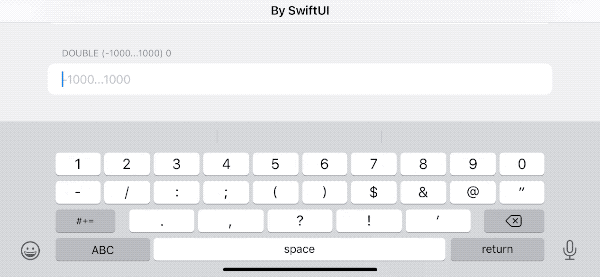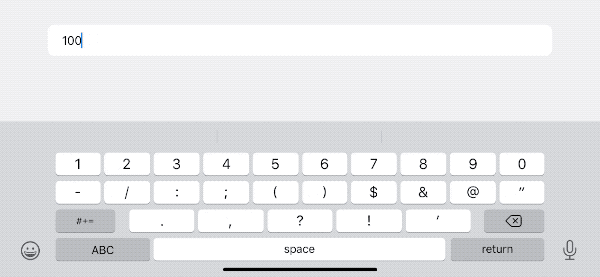# SwiftUI TextField 进阶——格式与校验

SwiftUI 的 TextField 可能是开发者在应用程序中最常使用的文本录入组件了。作为 UITextField（NSTextField）的 SwiftUI 封装，苹果为开发者提供了众多的构造方法和修饰符以提高其使用的便利性、定制性。但 SwiftUI 在封装中也屏蔽了不少的高级接口和功能，增加了开发者实现某些特定需要的复杂性。本文为【SwiftUI 进阶】系列文章中的一篇，在本文中，我将介绍如何在 TextField 中实现如下功能：

• 屏蔽无效字符
• 判断录入的内容是否满足特定条件
• 对录入的文本实时格式化显示## 为什么不自己封装新的实现

• 优先考虑能否在 SwiftUI 原生方法中找到解决手段
• 如确需采用非原生方法，尽量采用非破坏性的实现，新增功能不能以牺牲原有功能为代价（需兼容官方的 SwiftUI 修饰方法）

## 如何在 TextField 中实现格式化显示

### 现有格式化方法

``````struct FormatterDemo:View{
@State var number = 100
var body: some View{
Form{
TextField("inputNumber",value:\$number,format: .number)
}
}
}
``````### 可能的格式化解决思路

• 在录入过程中激活 TextField 内置的 Formatter，让其能够在文本发生变化时对内容进行格式化
• 在文本发生变化时调用自己实现的 Format 方法，对内容进行实时格式化

``````            TextField("inputNumber",value:\$number,format: .number)
.introspectTextField{ td in
td.delegate = nil
}
``````

## 如何在 TextField 中屏蔽无效字符

### 现有屏蔽字符方法

``````TextField("inputNumber",value:\$number,format: .number)
``````

• 只支持部分类型的设备
• 支持的键盘类型有限

### 可能的屏蔽字符解决思路

• 使用 UITextFieldDelegate 的`textField`方法
• 在 SwiftUI 的视图中，使用`onChange`在录入发生变化时进行判断并修改

``````func textField(_ textField: UITextField, shouldChangeCharactersIn range: NSRange, replacementString string: String) -> Bool {
// 检查 string 是否满足条件
if 满足条件 { return true } // 将新增字符添加到输入框
else { return false}
}
``````

## 如何在 TextField 中检查内容是否符合指定条件

``````TextField("inputNumber", value: \$number, format: .number)
.foregroundColor(number < 100 ? .red : .primary)
``````

## 其他需要注意的问题

### 本地化

``````1,000,000.012 // 大多数地区
1 000 000,012 // fr
``````

### Formatter

SwiftUI 的 TextField 目前对新老两种 Formatter 都提供了对应的构造方法。我倾向于使用新的 Formatter API。其为旧 Formatter API 的 Swift 原生实现，提供了更便捷、更安全的的声明方式。关于新 Formatter 的更多资料请阅读 WWDC 2021 新 Formatter API：新老比较及如何自定义

``````@State var number = 100
TextField("inputNumber", value: \$number, format: .number)
``````

### 易用性

``````// 方案一
let intDelegate = ValidationDelegate(type: .int, maxLength: 6)

TextField("0...1000", value: \$intValue, format: .number)
.numberValidator(value: intValue) { \$0 < 0 || \$0 > 1000 }

// 方案二
@StateObject var intStore = NumberStore(text: "",
type: .int,
maxLength: 5,
allowNagative: true,
formatter: IntegerFormatStyle<Int>())

TextField("-1000...1000", text: \$intStore.text)
.formatAndValidate(intStore) { \$0 < -1000 || \$0 > 1000 }
``````

## 方案一

``````public init<F>(_ titleKey: LocalizedStringKey, value: Binding<F.FormatInput>, format: F, prompt: Text? = nil) where F : ParseableFormatStyle, F.FormatOutput == String
``````

``````func textField(_ textField: UITextField, shouldChangeCharactersIn range: NSRange, replacementString string: String) -> Bool {
let text = textField.text ?? ""
return validator(text: text, replacementString: string)
}

private func validator(text: String, replacementString string: String) -> Bool {
// 判断有效字符
guard string.allSatisfy({ characters.contains(\$0) }) else { return false }
let totalText = text + string

// 检查小数点
if type == .double, text.contains(decimalSeparator), string.contains(decimalSeparator) {
return false
}

// 检查负号
let minusCount = totalText.components(separatedBy: minusCharacter).count - 1

if minusCount > 1 {
return false
}
if minusCount == 1, !totalText.hasPrefix("-") {
return false
}

// 检查长度
guard totalText.count < maxLength + minusCount else {
return false
}
return true
}
``````

``````extension View {
// 根据是否满足指定条件调整文字颜色
func numberValidator<T: Numeric>(value: T, errorCondition: (T) -> Bool) -> some View {
foregroundColor(errorCondition(value) ? .red : .primary)
}
// 替换 delegate
func addTextFieldDelegate(delegate: UITextFieldDelegate) -> some View {
introspectTextField { td in
td.delegate = delegate
}
}
}
``````

## 方案二

``````class NumberStore<T: Numeric, F: ParseableFormatStyle>: ObservableObject where F.FormatOutput == String, F.FormatInput == T {
@Published var text: String
let type: ValidationType
let maxLength: Int
let allowNagative: Bool
private var backupText: String
var error: Bool = false
private let locale: Locale
let formatter: F

init(text: String = "",
type: ValidationType,
maxLength: Int = 18,
allowNagative: Bool = false,
formatter: F,
locale: Locale = .current)
{
self.text = text
self.type = type
self.allowNagative = allowNagative
self.formatter = formatter
self.locale = locale
backupText = text
self.maxLength = maxLength == .max ? .max - 1 : maxLength
}
``````

formatter 传递给`NumberStore`，并在`getValue`中调用。

``````// 返回验证后的数字
func getValue() -> T? {
// 特殊处理（无内容、只有负号、浮点数首字母为小数点）
if text.isEmpty || text == minusCharacter || (type == .double && text == decimalSeparator) {
backup()
return nil
}

// 用去除组分隔符后的字符串判断字符是否有效
let pureText = text.replacingOccurrences(of: groupingSeparator, with: "")
guard pureText.allSatisfy({ characters.contains(\$0) }) else {
restore()
return nil
}

// 处理多个小数点情况
if type == .double {
if text.components(separatedBy: decimalSeparator).count > 2 {
restore()
return nil
}
}

// 多个负号情况
if minusCount > 1 {
restore()
return nil
}

// 负号必须为首字母
if minusCount == 1, !text.hasPrefix("-") {
restore()
return nil
}

// 判断长度
guard text.count < maxLength + minusCount else {
restore()
return nil
}

// 将文字转换成数字，然后再转换为文字（保证文字格式正确）
if let value = try? formatter.parseStrategy.parse(text) {
let hasDecimalCharacter = text.contains(decimalSeparator)
text = formatter.format(value)
// 保护最后的小数点（不特别处理的话，转换回来的文字可能不包含小数点）
if hasDecimalCharacter, !text.contains(decimalSeparator) {
text.append(decimalSeparator)
}
backup()
return value
} else {
restore()
return nil
}
}
``````

View 扩展

``````extension View {
@ViewBuilder
func formatAndValidate<T: Numeric, F: ParseableFormatStyle>(_ numberStore: NumberStore<T, F>, errorCondition: @escaping (T) -> Bool) -> some View {
onChange(of: numberStore.text) { text in
if let value = numberStore.getValue(),!errorCondition(value) {
numberStore.error = false // 通过 NumberStore 转存校验状态
} else if text.isEmpty || text == numberStore.minusCharacter {
numberStore.error = false
} else { numberStore.error = true }
}
.foregroundColor(numberStore.error ? .red : .primary)
.disableAutocorrection(true)
.autocapitalization(.none)
.onSubmit { // 处理只有一个小数点的情况
if numberStore.text.count > 1 && numberStore.text.suffix(1) == numberStore.decimalSeparator {
numberStore.text.removeLast()
}
}
}
}
``````

## 两种方案的比较

• 效率

• 支持的类型种类

• 可选值支持

• 可持续性（SwiftUI 向后兼容性）

• 对其他修饰方法的兼容性

## 总结

 聊聊两年来我使用【健康笔记】的体会以及近几年的身体状况 AttributedString——不仅仅让文字更漂亮